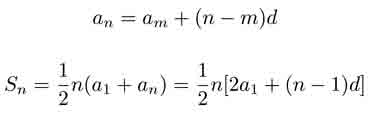SEARCH HOMEMath Central Quandaries & QueriesQuestion from Alice, a student: Find the 24th term of this sequence 6.8,8.0,9.2... Find the sum of the first 21 termsHi Alice,

The sequence is arithmetic so you can use the following formulae:an nth term in the sequence am mth term in the sequence d difference between consecutive terms n, m term position in sequence (1st, 2nd, 3rd, etc.) a1 first term in the sequence Sn sum of the first n terms

Hope this helps,

JaniceMath Central is supported by the University of Regina and The Pacific Institute for the Mathematical Sciences.